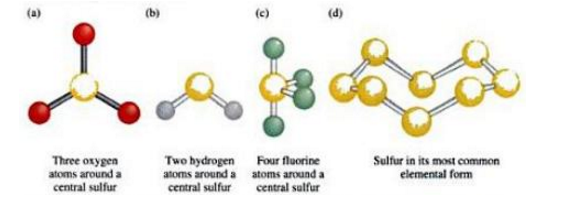# Chemical Formula

Chemical Formula

The Chemical Formula is a clue to the makeup of a compound regarding the types of atoms and their relative numeric. From the understanding of oxidation numbers and valence and a knowledge of atomic structure, it is possible to write chemical formulas.A chemical formula is a “notation made up of the chemical symbols of the elements present in a compound and numerical subscript that indicate the number of atoms of each element present in a molecule of the compound.”A chemical formula provides information about the atomic composition of a substance.

Solved Examples

Problem 1: In one molecule of the compound, determine how many atoms of every element are present for each one of these chemical formulas.

1.       HCN – hydrogen cyanide –It’s a toxic gas
2.       C18H21NO3 – codeine, a painkilling drug
3.       Ca10(PO4)6(OH)2 – Hydroxyapatite, that is present in the enamel of tooth

1. One atom is present in each of the elements hydrogen, carbon and nitrogen, respectively. Remember that the subscript 1 is understood when no subscript is mentioned.
2. This formula points out that in one molecule of the compound, 18 carbon atoms, 21 hydrogen atoms, one nitrogen atom and three oxygen atoms are existent.
3. There are ten calcium atoms. The amounts of phosphorous, hydrogen and oxygen are affected by the subscripts outside the parentheses. There are six phosphorous atoms and two hydrogen atoms existent. Oxygen atoms exist in two positions in the formula. There are overall 26 oxygen atoms: two from the OH subunit (2×1) and 24 from the PO4 sub units (6×4).Problem 2: Note the accurate chemical formulas for the compounds containing sulfur whose molecular images are as following.

SolutionSpecific guidelines must be followed when writing Chemical Formulas. One must make use of the correct subscript and symbols and arrange the symbols in the right order.

• Sulfur and Oxygen are in same group of the periodic table. Conferring to the third guideline, the lower element are arranged first. Therefore, SO3 is the exact formula.
• This compound comprises of sulfur and hydrogen elements from the Group 16 of the periodic table. Conferring to the second guideline, hydrogen will be arranged before elements from this group. Thus, H2S is the exact formula.
• The elements in this compound fall under two unlike groups of the periodic table. Conferring to the primary guideline, the element farther to the left arranged first. Therefore, SF4 is the exact formula.
• Just one element is present in this substance, whose molecules contain eight atoms. Thus, the correct formula is S8.

#### Practise This Question

The compound in which carbon uses only its sp3 hybrid orbitals for bond formation is?